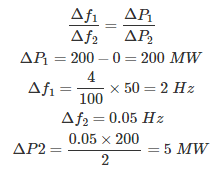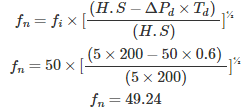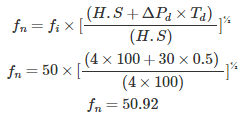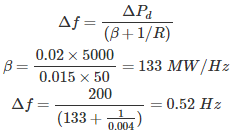# MCQs on Power Systems

##### Page 25 of 67. Go to page 1 2 3 4 5 6 7 8 9 10 11 12 13 14 15 16 17 18 19 20 21 22 23 24 25 26 27 28 29 30 31 32 33 34 35 36 37 38 39 40 41 42 43 44 45 46 47 48 49 50 51 52 53 54 55 56 57 58 59 60 61 62 63 64 65 66 67
01․ The advantage of hydro electric power station over thermal power station is
the initial cost of hydro electric power station is low
the operation cost of hydro electric power station is low
hydro electric power station can supply the power throughout the year
hydro electric station can be constructed at the place where the energy is required

Hydro electric power station do not require fuel like thermal power stations. Therefore operating cost of hydro electric power station is low. But initial cost is high, because to construct dam and for heavy equipment it requires high initial cost.

02․ In order to have lower cost of power generaion
the load factor and diversity factor should be low
The load factor and diversity factor should be high
the load factor should be low but diversity factor should be high
the load factor should be high but diversity factor should be low

Plant load factor is defined as the ratio of average power generation to the maximum power generation. Plant load factor = Average power/ Maximum power Diversity factor is defined as the ratio of sum of individual maximum demand to the station maximum demand. Diversity factor = Sum of individual maximum demand/ Station maximum demand. Therefore, both load factor and diversity factors are inversely proportional to maximum demand. If load factor and diversity factors are high, maximum demand will be less, it will reduce the machine rating and lower cost of power generation is possible.

03․ A 200 MW capacity generator has a speed regulation of 4% for a frequency drop of 0.05 Hz. Find the power input supplied by the turbine for maintaining constant frequency?
10 MW
5 MW
15 MW
20 MW

For maintaining constant frequencyΔf = Change in frequency ΔP = Change in power input

04․ A 200 MVA alternator operated at no load at frequency of 50 Hz, it has inertia constant of 5 MWsec/MVA and governing system has a time delay of 0.6 sec. If the load of 50 MW is suddenly applied then find the frequency deviation during this time?
0.76 Hz
-0.76 Hz
0.85 Hz
-0.85 Hz

Frequency deviation due to time delay in the governing system: Due to sudden load demand Where, fn = New frequency fi = Initial frequency ΔPd = Load demand S = Rating of generator Td = Time delay in governing system H = Inertia constant of generatorFrequency deviation Δf = fn - fi Frequency deviation Δf = 50 - 49.24 = -0.76 Hz

05․ A 100 MVA alternator operating at full load at 50 Hz. H = 4 MWsec/MVA, Td = 0.5 sec. One of the transmission line is tripped. So that output of generator is reduced to 70 MW. Find the frequency deviation during this time?
0.56
-0.56
0.92
-0.92

Frequency deviation due to time delay in the governing system: Due to sudden load loss Where, fn = New frequency fi = Initial frequency ΔPd = Load demand S = Rating of generator Td = Time delay in governing system H = Inertia constant of generatorFrequency deviation Δf = fn - fi Frequency deviation Δf = 50.92 - 50 = 0.92

06․ In which of the following frequency control method tie line may be overloaded?
flat frequency control
flat tie line control
parallel frequency
all of the above

Flat frequency control: Load changes of one bus bar is supplied by the another generator for maintaining constant frequency through the tie line. In this method tie line may be overloaded. Flat tie line control: In this method tie line power flow is maintained constant without overload of tie line. The control signal of each area proportional to changes in the frequency and changes in the tie line power. Parallel frequency control: It is the most practical method in which both the generators simultaneously respond for the load changes in either of the bus bars.

07․ The equation of area control error(ACE) is
ACE = ΔPtie + b.Δf
ACE = ΔPtie - b.Δf
ACE = ΔPtie +1/ b.Δf
ACE = ΔPtie - 1/b.Δf

The equation of area control error(ACE) = ΔPtie + b.Δf Where, ΔPtie = Power change in tie line Δf = Change in frequency b = Bias constant = -1/R R = Speed regulation coefficient

08․ Power system network has a total power of 5000 MW at 50 Hz supplying to the different consumers. Frequency is varying at 1.5% per change of 2% load. Assume R =0.004 Hz/MW. Find the steady state frequency deviation if a sudden loss of 200 MW occur due to tripping of one of the transmission line?
50 Hz
0.52 Hz
0.48 Hz
-0.52 Hz

Steady state frequency drop due to sudden loss Δf = ΔPd/(β + 1/R) Where, ΔPd = Load demand β = % change in load/ % change in frequency R = Speed regulation constant09․ Units of incremental cost is
Rs/hr
RsMW/hr
Rs/MWhr
MWhr/Rs

Relation between fuel consumption and power F = a + b P + C P² Where, a,b and c are constants P = Power in MW F = Fuel consumption in Rs/hr Incremental cost = dF/dP = b + 2CP Rs/MWhr

10․ Two generating stations delivering a powers of P1 = 150 MW, P2 =100 MW and the B coefficients are B11 = 0.005, B22 = 0.002, B12=B21= -0.001. Find the transmission line losses?
85.5
95.5
102.5
105.2

Power loss of a transmission line PL = B11×P1² + B22×P2² + 2×B12×P1×P2 Where, B11,B22,B12 and B21 are B coefficients P1 and P2 are power delivered by generating stations. PL = 0.005×150² + 0.002×100² +2×(-0.002)×150×100 PL = 102.5 MW

<<<2324252627>>>